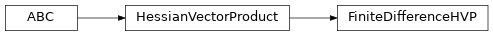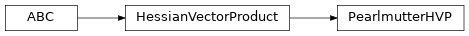# `garage.tf.optimizers`¶

TensorFlow optimizers.

class ConjugateGradientOptimizer(cg_iters=10, reg_coeff=1e-05, subsample_factor=1.0, backtrack_ratio=0.8, max_backtracks=15, accept_violation=False, hvp_approach=None, num_slices=1)

Performs constrained optimization via line search.

The search direction is computed using a conjugate gradient algorithm, which gives x = A^{-1}g, where A is a second order approximation of the constraint and g is the gradient of the loss function.

Parameters
• cg_iters (int) – The number of CG iterations used to calculate A^-1 g

• reg_coeff (float) – A small value so that A -> A + reg*I

• subsample_factor (float) – Subsampling factor to reduce samples when using “conjugate gradient. Since the computation time for the descent direction dominates, this can greatly reduce the overall computation time.

• backtrack_ratio (float) – backtrack ratio for backtracking line search.

• max_backtracks (int) – Max number of iterations for backtrack linesearch.

• accept_violation (bool) – whether to accept the descent step if it violates the line search condition after exhausting all backtracking budgets.

• hvp_approach (HessianVectorProduct) – A class that computes Hessian-Vector products.

• num_slices (int) – Hessian-vector product function’s inputs will be divided into num_slices and then averaged together to improve performance.

update_opt(loss, target, leq_constraint, inputs, extra_inputs=None, name='ConjugateGradientOptimizer', constraint_name='constraint')

Update the optimizer.

Build the functions for computing loss, gradient, and the constraint value.

Parameters
• loss (tf.Tensor) – Symbolic expression for the loss function.

• target (garage.tf.policies.Policy) – A parameterized object to optimize over.

• leq_constraint (tuple[tf.Tensor, float]) – A constraint provided as a tuple (f, epsilon), of the form f(*inputs) <= epsilon.

• inputs (list(tf.Tenosr)) – A list of symbolic variables as inputs, which could be subsampled if needed. It is assumed that the first dimension of these inputs should correspond to the number of data points.

• extra_inputs (list[tf.Tenosr]) – A list of symbolic variables as extra inputs which should not be subsampled.

• name (str) – Name to be passed to tf.name_scope.

• constraint_name (str) – A constraint name for prupose of logging and variable names.

loss(inputs, extra_inputs=None)

Compute the loss value.

Parameters
• inputs (list[numpy.ndarray]) – A list inputs, which could be subsampled if needed. It is assumed that the first dimension of these inputs should correspond to the number of data points

• extra_inputs (list[numpy.ndarray]) – A list of extra inputs which should not be subsampled.

Returns

Loss value.

Return type

float

constraint_val(inputs, extra_inputs=None)

Constraint value.

Parameters
• inputs (list[numpy.ndarray]) – A list inputs, which could be subsampled if needed. It is assumed that the first dimension of these inputs should correspond to the number of data points

• extra_inputs (list[numpy.ndarray]) – A list of extra inputs which should not be subsampled.

Returns

Constraint value.

Return type

float

optimize(inputs, extra_inputs=None, subsample_grouped_inputs=None, name='optimize')

Optimize the function.

Parameters
• inputs (list[numpy.ndarray]) – A list inputs, which could be subsampled if needed. It is assumed that the first dimension of these inputs should correspond to the number of data points

• extra_inputs (list[numpy.ndarray]) – A list of extra inputs which should not be subsampled.

• subsample_grouped_inputs (list[numpy.ndarray]) – Subsampled inputs to be used when subsample_factor is less than one.

• name (str) – The name argument for tf.name_scope.

class FiniteDifferenceHVP(base_eps=1e-08, symmetric=True, num_slices=1)

Bases: `HessianVectorProduct`Computes Hessian-vector product using finite difference method.

Parameters
• base_eps (float) – Base epsilon value.

• symmetric (bool) – Symmetric or not.

• num_slices (int) – Hessian-vector product function’s inputs will be divided into num_slices and then averaged together to improve performance.

update_hvp(f, target, inputs, reg_coeff, name='FiniteDifferenceHVP')

Build the symbolic graph to compute the Hessian-vector product.

Parameters
• f (tf.Tensor) – The function whose Hessian needs to be computed.

• target (garage.tf.policies.Policy) – A parameterized object to optimize over.

• inputs (tuple[tf.Tensor]) – The inputs for function f.

• reg_coeff (float) – A small value so that A -> A + reg*I.

• name (str) – Name to be used in tf.name_scope.

build_eval(inputs)

Build the evaluation function. # noqa: D202, E501 # https://github.com/PyCQA/pydocstyle/pull/395.

Parameters

inputs (tuple[numpy.ndarray]) – Function f will be evaluated on these inputs.

Returns

It can be called to get the final result.

Return type

function

class PearlmutterHVP(num_slices=1)

Bases: `HessianVectorProduct`Computes Hessian-vector product using Pearlmutter’s algorithm.

`Pearlmutter, Barak A. “Fast exact multiplication by the Hessian.” Neural

computation 6.1 (1994): 147-160.`

update_hvp(f, target, inputs, reg_coeff, name='PearlmutterHVP')

Build the symbolic graph to compute the Hessian-vector product.

Parameters
• f (tf.Tensor) – The function whose Hessian needs to be computed.

• target (garage.tf.policies.Policy) – A parameterized object to optimize over.

• inputs (tuple[tf.Tensor]) – The inputs for function f.

• reg_coeff (float) – A small value so that A -> A + reg*I.

• name (str) – Name to be used in tf.name_scope.

build_eval(inputs)

Build the evaluation function. # noqa: D202, E501 # https://github.com/PyCQA/pydocstyle/pull/395.

Parameters

inputs (tuple[numpy.ndarray]) – Function f will be evaluated on these inputs.

Returns

It can be called to get the final result.

Return type

function

class FirstOrderOptimizer(optimizer=None, learning_rate=None, max_optimization_epochs=1000, tolerance=1e-06, batch_size=32, callback=None, verbose=False, name='FirstOrderOptimizer')

First order optimier.

Parameters
• optimizer (tf.Optimizer) – Optimizer to be used.

• learning_rate (dict) – learning rate arguments. learning rates are our main interest parameters to tune optimizers.

• max_optimization_epochs (int) – Maximum number of epochs for update.

• tolerance (float) – Tolerance for difference in loss during update.

• batch_size (int) – Batch size for optimization.

• callback (callable) – Function to call during each epoch. Default is None.

• verbose (bool) – If true, intermediate log message will be printed.

• name (str) – Name scope of the optimizer.

update_opt(loss, target, inputs, extra_inputs=None, **kwargs)

Construct operation graph for the optimizer.

Parameters
• loss (tf.Tensor) – Loss objective to minimize.

• target (object) – Target object to optimize. The object should implemenet get_params() and get_param_values.

• inputs (list[tf.Tensor]) – List of input placeholders.

• extra_inputs (list[tf.Tensor]) – List of extra input placeholders.

• kwargs (dict) – Extra unused keyword arguments. Some optimizers have extra input, e.g. KL constraint.

loss(inputs, extra_inputs=None)

The loss.

Parameters
• inputs (list[numpy.ndarray]) – List of input values.

• extra_inputs (list[numpy.ndarray]) – List of extra input values.

Returns

Loss.

Return type

float

Raises

Exception – If loss function is None, i.e. not defined.

optimize(inputs, extra_inputs=None, callback=None)

Perform optimization.

Parameters
• inputs (list[numpy.ndarray]) – List of input values.

• extra_inputs (list[numpy.ndarray]) – List of extra input values.

• callback (callable) – Function to call during each epoch. Default is None.

Raises
class LBFGSOptimizer(max_opt_itr=20, callback=None)

Limited-memory BFGS (L-BFGS) optimizer.

Performs unconstrained optimization via L-BFGS.

Parameters
• max_opt_itr (int) – Maximum iteration for update.

• callback (callable) – Function to call during optimization.

update_opt(loss, target, inputs, extra_inputs=None, name='LBFGSOptimizer', **kwargs)

Construct operation graph for the optimizer.

Parameters
• loss (tf.Tensor) – Loss objective to minimize.

• target (object) – Target object to optimize. The object should implemenet get_params() and get_param_values.

• inputs (list[tf.Tensor]) – List of input placeholders.

• extra_inputs (list[tf.Tensor]) – List of extra input placeholders.

• name (str) – Name scope.

• kwargs (dict) – Extra unused keyword arguments. Some optimizers have extra input, e.g. KL constraint.

loss(inputs, extra_inputs=None)

The loss.

Parameters
• inputs (list[numpy.ndarray]) – List of input values.

• extra_inputs (list[numpy.ndarray]) – List of extra input values.

Returns

Loss.

Return type

float

Raises

Exception – If loss function is None, i.e. not defined.

optimize(inputs, extra_inputs=None, name='optimize')

Perform optimization.

Parameters
• inputs (list[numpy.ndarray]) – List of input values.

• extra_inputs (list[numpy.ndarray]) – List of extra input values.

• name (str) – Name scope.

Raises

Exception – If loss function is None, i.e. not defined.

class PenaltyLBFGSOptimizer(max_opt_itr=20, initial_penalty=1.0, min_penalty=0.01, max_penalty=1000000.0, increase_penalty_factor=2, decrease_penalty_factor=0.5, max_penalty_itr=10, adapt_penalty=True)

Penalized Limited-memory BFGS (L-BFGS) optimizer.

Performs constrained optimization via penalized L-BFGS. The penalty term is adaptively adjusted to make sure that the constraint is satisfied.

Parameters
• max_opt_itr (int) – Maximum iteration for update.

• initial_penalty (float) – Initial penalty.

• min_penalty (float) – Minimum penalty allowed. Penalty will be clipped if lower than this value.

• max_penalty (float) – Maximum penalty allowed. Penalty will be clipped if higher than this value.

• increase_penalty_factor (float) – Factor to increase penalty in each penalty iteration.

• decrease_penalty_factor (float) – Factor to decrease penalty in each penalty iteration.

• max_penalty_itr (int) – Maximum penalty iterations to perform.

• adapt_penalty (bool) – Whether the penalty is adaptive or not. If false, penalty will not change.

update_opt(loss, target, leq_constraint, inputs, constraint_name='constraint', name='PenaltyLBFGSOptimizer', **kwargs)

Construct operation graph for the optimizer.

Parameters
• loss (tf.Tensor) – Loss objective to minimize.

• target (object) – Target object to optimize. The object should implemenet get_params() and get_param_values.

• leq_constraint (tuple) – It contains a tf.Tensor and a float value. The tf.Tensor represents the constraint term, and the float value is the constraint value.

• inputs (list[tf.Tensor]) – List of input placeholders.

• constraint_name (str) – Constraint name for logging.

• name (str) – Name scope.

• kwargs (dict) – Extra unused keyword arguments. Some optimizers have extra input, e.g. KL constraint.

loss(inputs)

The loss.

Parameters

inputs (list[numpy.ndarray]) – List of input values.

Returns

Loss.

Return type

float

Raises

Exception – If loss function is None, i.e. not defined.

constraint_val(inputs)

The constraint value.

Parameters

inputs (list[numpy.ndarray]) – List of input values.

Returns

Constraint value.

Return type

float

Raises

Exception – If loss function is None, i.e. not defined.

optimize(inputs, name='optimize')

Perform optimization.

Parameters
• inputs (list[numpy.ndarray]) – List of input values.

• name (str) – Name scope.

Raises

Exception – If loss function is None, i.e. not defined.